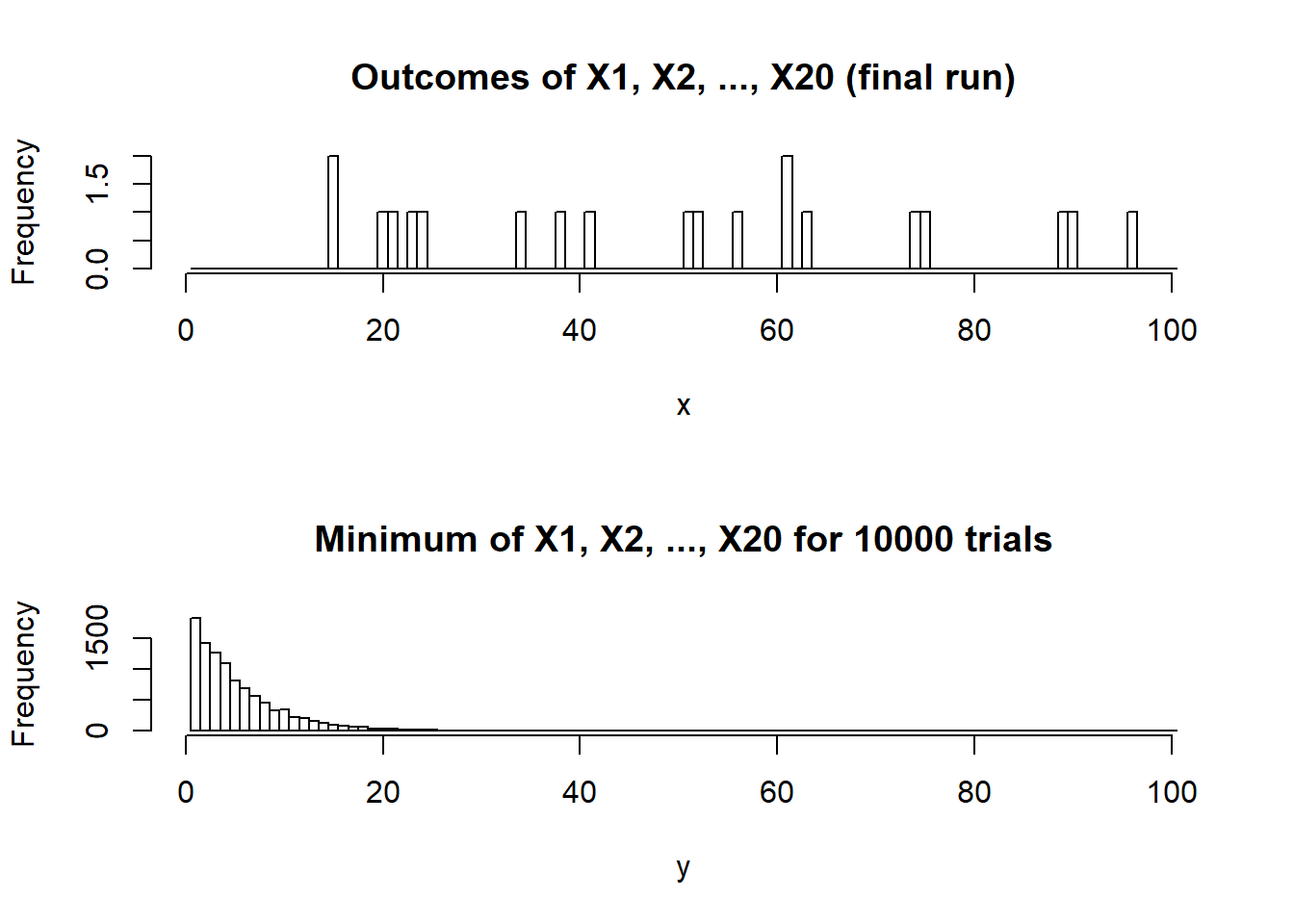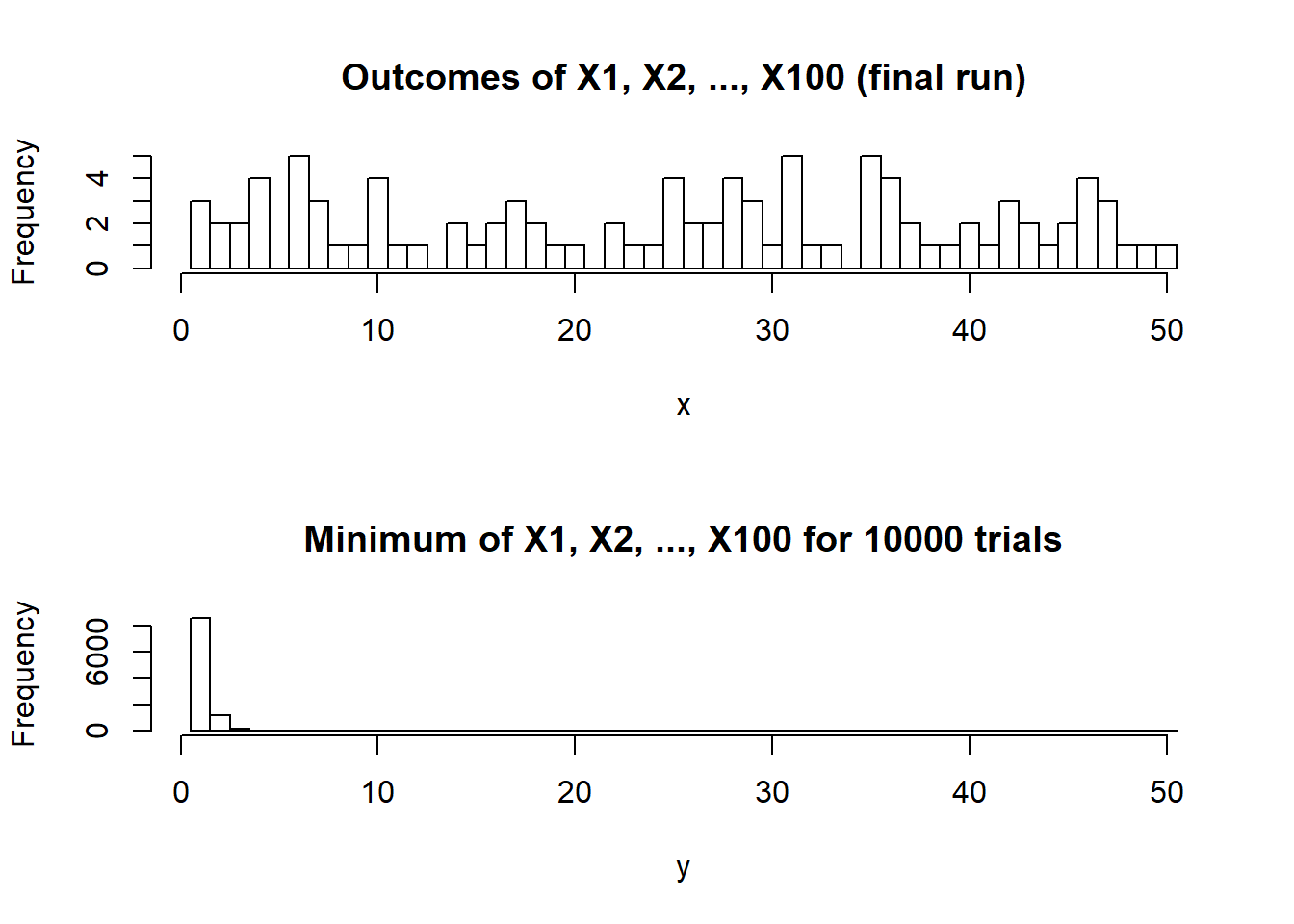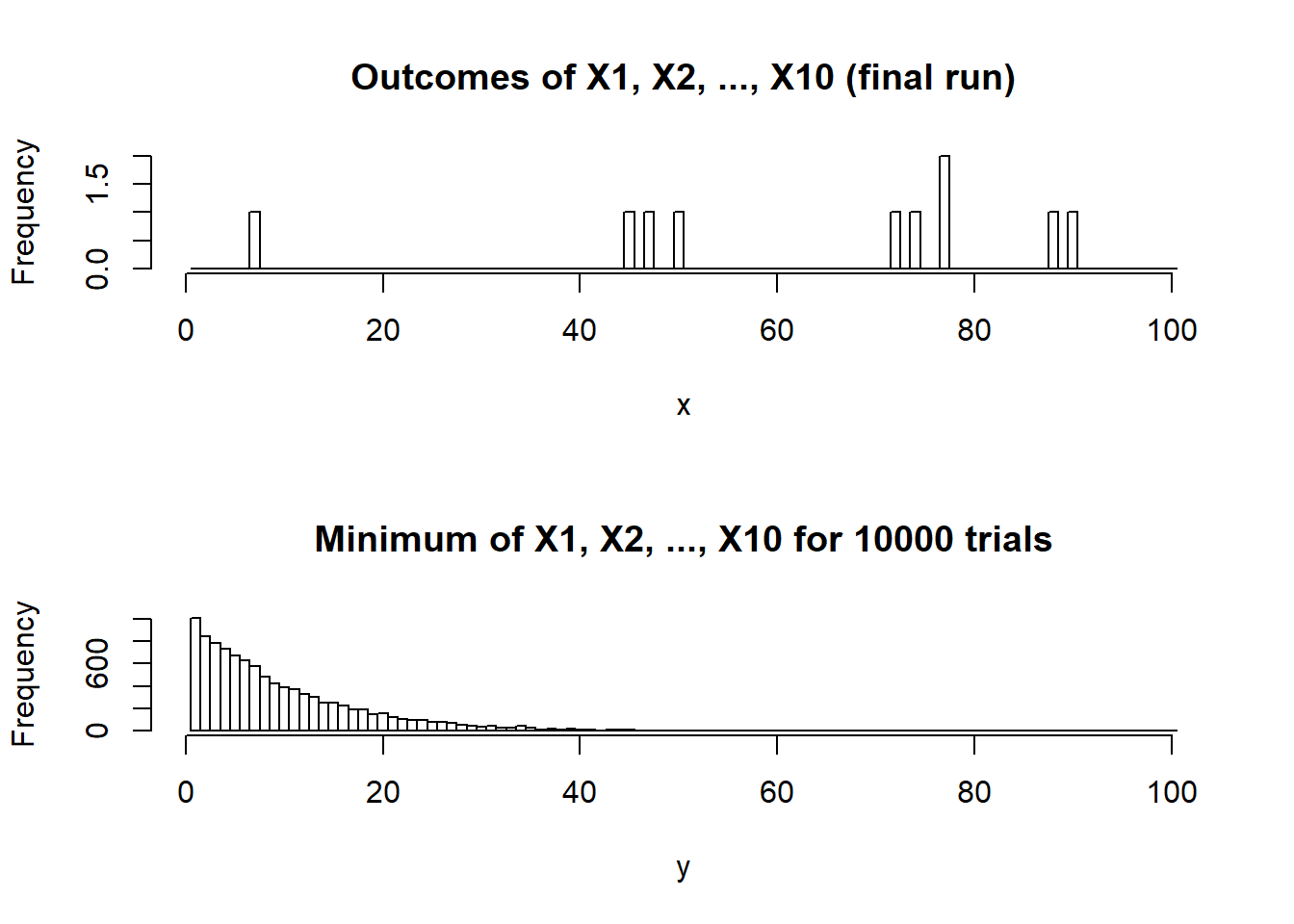# Probability Distributions

## Question 1

Let X1, X2, . . . , Xn be n mutually independent random variables, each of which is uniformly distributed on the integers from 1 to k. Let Y denote the minimum of the Xi’s. Find the distribution of Y .

### Theoretical

$F(Y = min) = (\frac{possible outcomes}{tot. outcomes})^n$ In the folling, k^2 represents the total possible outcomes, and k^n - (k-1)^n represents outcomes where at least one independent random variable is equal to 1.

$F(Y = 1) = \frac{k^n - (k-1)^n}{k^n}$

In the folling, k^2 represents the total possible outcomes, and (k-1)^n - (k-2)^n represents outcomes where at least one independent random variable is equal to 2 and no independent random variable is equal to 1. $F(Y = 2) = \frac{(k-1)^n - (k-2)^n}{k^n}$

In the folling, k^2 represents the total possible outcomes, and (k-2)^n - (k-3)^n represents outcomes where at least one independent random variable is equal to 3 and no independent random variable is equal to 1 or 2. $F(Y = 3) = \frac{(k-2)^n - (k-3)^n}{k^n}$

General solution:

$F(Y = min) = \frac{(k-min+1)^n - (k-min)^n}{k^n}$

### Simulation

Simulated distributions are plotted below.

#### 20 mutually independent random variables, values between 1 and 100

n <- 20 # set value of n - number of mutually independent random variables
k <- 100 # set value of k
min <- 1
max <- k

y <- c()
y_trials <- 10000
for (i in (1:y_trials)){
x <- as.integer(runif(n,min,max+1) )        #generate random uniform numcases numbers from min to max
y <- append(y,min(x))
i = i+1
}

#as.integer truncates, round converts to integers, add .5 for equal intervals
par(mfrow=c(2,1))                        #stack two figures above each other
hist(x,main=paste0("Outcomes of X1, X2, ..., X", n, " (final run)"),breaks=seq(min-.5,max+.5,1))          #show the histogram

hist(y,main=paste0("Minimum of X1, X2, ..., X", n, " for ", y_trials, " trials"),breaks=seq(min-.5,max+.5,1))          #show the histogram#### 100 mutually independent random variables, values between 1 and 50

n <- 100 # set value of n - number of mutually independent random variables
k <- 50 # set value of k
min <- 1
max <- k

y <- c()
y_trials <- 10000
for (i in (1:y_trials)){
x <- as.integer(runif(n,min,max+1) )        #generate random uniform numcases numbers from min to max
y <- append(y,min(x))
i = i+1
}

#as.integer truncates, round converts to integers, add .5 for equal intervals
par(mfrow=c(2,1))                        #stack two figures above each other
hist(x,main=paste0("Outcomes of X1, X2, ..., X", n, " (final run)"),breaks=seq(min-.5,max+.5,1))          #show the histogram

hist(y,main=paste0("Minimum of X1, X2, ..., X", n, " for ", y_trials, " trials"),breaks=seq(min-.5,max+.5,1))          #show the histogram#### 10 mutually independent random variables, values between 1 and 100

n <- 10 # set value of n - number of mutually independent random variables
k <- 100 # set value of k
min <- 1
max <- k

y <- c()
y_trials <- 10000
for (i in (1:y_trials)){
x <- as.integer(runif(n,min,max+1) )        #generate random uniform numcases numbers from min to max
y <- append(y,min(x))
i = i+1
}

#as.integer truncates, round converts to integers, add .5 for equal intervals
par(mfrow=c(2,1))                        #stack two figures above each other
hist(x,main=paste0("Outcomes of X1, X2, ..., X", n, " (final run)"),breaks=seq(min-.5,max+.5,1))          #show the histogram

hist(y,main=paste0("Minimum of X1, X2, ..., X", n, " for ", y_trials, " trials"),breaks=seq(min-.5,max+.5,1))          #show the histogram## Question 2

Your organization owns a copier (future lawyers, etc.) or MRI (future doctors). This machine has a manufacturer’s expected lifetime of 10 years. This means that we expect one failure every ten years. (Include the probability statements and R Code for each part.).

### Part A

1. What is the probability that the machine will fail after 8 years?. Provide also the expected value and standard deviation. Model as a geometric. (Hint: the probability is equivalent to not failing during the first 8 years..)

Geometric - the number of trials needed to get one success. Consider a success to be the machine failing. The expected value p = p(maching failing each year) = 0.1 q = 1 - p = 0.9

#### Probability

Geometric cdf that the machine will not fail in the first 8 years: $Prob = (q)^k = (0.9)^8 = 0.43046721$

#### Expected Value

Expected value = expected no. years until machine failure

$E(x) = \frac{1}{p} = \frac{1}{0.1} = 10 years$

#### Standard Deviation

$Stdev = \sqrt{\frac{1-p}{p^2}} = \sqrt{\frac{1-0.1}{0.1^2}} = 9.487$

### Part B

1. What is the probability that the machine will fail after 8 years?. Provide also the expected value and standard deviation. Model as an exponential.

#### Probability

Exponential - the amount of time until an event occurs. $Prob = e^{-\lambda x} = e^{-0.1 * 8} = 0.44932896411$

#### Expected Value

Expected value = expected no. years until machine failure

$E(x) = \frac{1}{\lambda} = \frac{1}{0.1} = 10 years$

#### Standard Deviation

$Stdev = \frac{1}{\lambda} = \frac{1}{0.1} = 10$

### Part C

1. What is the probability that the machine will fail after 8 years?. Provide also the expected value and standard deviation. Model as a binomial. (Hint: 0 success in 8 years)

#### Probability

Binomial - the number of successes in a sequence of n independent true/false events

$Prob = (^n_i)p^i(1-p)^{n-i} = (^8_0)0.1^0(0.9)^{8-0} = 0.9^8 = 0.4304672$

#### Expected Value

$E(x) = np = 8 * 0.1 = 0.8$

#### Standard Deviation

$Stdev = \sqrt{np(1-p)} = \sqrt{0.8(0.9)} = 0.84852813742$

### Part D

1. What is the probability that the machine will fail after 8 years?. Provide also the expected value and standard deviation. Model as a Poisson.

#### Probability

Poisson - probability of number of events occuring in period of time

$prob = \frac{\lambda^k}{k!}*e^{-\lambda} = \frac{0.8^0}{0!}*e^{-0.8} = 0.44932896411$

#### Expected Value

$E(x) = \lambda = 0.8$

#### Standard Deviation

$Stdev = \sqrt{\lambda} = \sqrt{0.8} = 0.894427191$